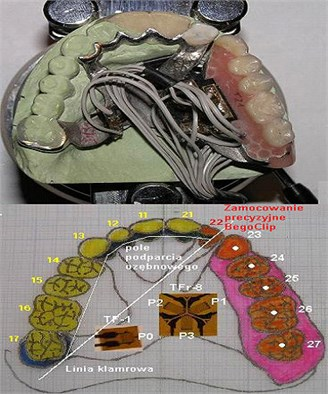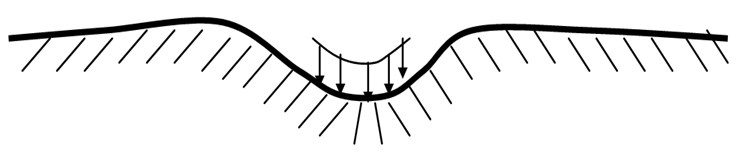Published: 26 September 2017

# Modeling of the denture’s elastic base

Marek Kuchta1
Rafał Białek2
Jacek Paś3
1, 2, 3Military University of Technology, Faculty of Electronics, Warsaw, Poland
Corresponding Author:
Marek Kuchta
Views 28

#### Abstract

This article discusses problems during modeling of the denture’s elastic base. The basic simplifications adopted in the analysis allows the use of formulae taken from the theory of elasticity.

## 1. Introduction

The full mathematical description of the denture’s operation during chewing requires its consideration in terms of the entire biosystem and, hence, it must be supported by the model of the gum soft tissue’s resistance, with which it cooperates closely. No denture tests, especially strength tests but not only, are possible without taking into account its flexible support. The proposed model of the gum’s soft tissues is based on large simplifications that require the analysed prosthetic base, like other soft tissues of the other surrounding body areas, to be characterised with elasticity (flexibility) that involves, after the deformation effected by external forces (within certain, small limits), its return to the original shape when these forces cease to effect. Unfortunately, it is not a permanent feature because the factors causing the change of or even the loss of elasticity – e.g. oedemas, tissues dehydration, body’s ageing – are known. Moreover, it is stated that the soft tissues of the prosthetic base tend to lose elasticity over time of remaining without the prosthetic cover . During its operation, the denture should be supported by the palate’s mucous membrane (Fig. 1).

Fig. 1Graphical representation of the model of dentureThe basic assumption adopted in the analysis of the cooperation of the denture and mucous membrane’s media is the statement that the palate’s mucous membrane may be considered as the environment, at the calculation of which, under certain conditions, the formulae taken from the theory of elasticity may be applied. The primary condition, which must take place, is the elasticity of the mucous membrane. The opportunity to determine this elasticity must exist as well – this is another indispensable condition to meet.

Before the analysis of such a specified elastic ground, one must refer to some hypotheses thought for other elastic centres that may be applied here as well .

## 2. Hypotheses of ground compressibility

Hypotheses of ground coefficient It assumes that the settlement $\omega$ of any ground point is proportional to the pressure $p$ applied at this point, i.e. that:

1
$p=K\bullet \omega ,$

where: $K$ – ground coefficient.

As can be seen, according to this hypothesis, the settlement of a given point depends on the pressure applied at that point and it does not depend on the pressures operating nearby at all. This manner of ground operation can be compared with the operation of an assembly of unrelated springs.

This hypothesis is strongly simplified – hence the opinions prevailing about it concerning its very limited applicability, e.g. as “first approximation” at best. The basic difficulty in the practical application of this hypothesis is the impossibility to determine precisely the proportionality coefficient K between pressures and settlements. This coefficient depends not only on the physical properties of the palate tissue but also on other factors occurring at the time of its measurement (e.g. the size of the pressure item’s base) . Besides, contrary to the basic assumption, the membrane settles not only within the boundaries of the loaded area but in the immediate vicinity outside this area (Fig. 2).

Fig. 2Graphical representation of the hypothesis of compressibility modulusAnother hypothesis (it can be called the hypothesis of compressibility modulus) assumes that the palate’s soft tissue is a continuous, homogeneous, and elastic object, covering infinitely far down and from side to side, and from the top, it is limited by the plane . Such an object is called elastic half-space. The elastic properties of the object, according to this theory, is characterised with the compressibility (deformation) modulus ${E}_{0}$ and (to a lesser extent) the Poisson’s coefficient ${\nu }_{0}$.

However, it is necessary to remember about the difference existing between the mechanical properties of the palate’s mucous membrane and of a completely elastic body. Above all, the palate’s mucous membrane is subject to large inelastic deformations that often exceed the elastic deformations – hence, inter alia, the determination of ${E}_{0}$ as the compressibility modulus, not the elasticity modulus. Besides, at multiple loading the mucous membrane, the elastic deformation will be diminished over time and then the compressibility modulus ${E}_{0}$ will pass into the elasticity modulus $E$, whose volume is much greater .

The flow effect (passing into plastic state) observed in other similar materials effected by large loads that prevents the application of the theory of elasticity is rather unlikely to occur because the pain centre will not allow for such a great pressure .

## 3. Determining the calculated characteristics of the ground

In order to determine the compressibility modulus, it is necessary to have the diagram of the relationship between the settlements of the palate’s mucous membrane and the load. The compressibility modulus should be set within the limits of this part of the diagram, in which the settlement can still be regarded as proportional to the loads . Then, the following formula may be used:

2
$\frac{{E}_{0}}{1-{\nu }_{o}}=0,88·\frac{∆P}{∆s}\bullet \sqrt{F},$

where: $∆P$ – load increase, [N/mm2]; $∆s$ – settlement increase [mm]; $F$ – the surface of the pressure item tip [mm2]; ${\nu }_{0}$ – Poisson’s coefficient = 0,35-0,4.

In practice, one can encounter various special cases of the ground, clarifying the initial assumption that defines the elastic half-space. In this case, we also have to deal with the elastic ground with the shape deviating from the assumed one, as the layer that is difficult to deform covers within short distance from the surface. By approving this assumption, one can then, for calculations on the elastic ground, apply the hypothesis on the ground coefficient again. However, it is indispensable to learn earlier the compressibility coefficient E0 and the Poisson’s coefficient ${\nu }_{0}$. Only then it will be possible to determine the calculated value of the ground coefficient K as the average obtained from two following relationships:

a) in the absence of friction between the compressible layer and the underlayer:

3
$K=\frac{{E}_{0}}{1-{\nu }_{0}}.$

b) assuming that the compressible layer soft cannot move along the underlayer:

4
$K=\frac{\left(1-{\nu }_{0}\right)·{E}_{0}}{\left(1+{\nu }_{0}\right)·\left(1-2·{\nu }_{0}\right)·H},$

where: $H$ – layer thickness.

Additionally, if the thickness of the compressible layer is very low, it will be difficult to obtain the compressibility modulus values from the measurement tests. Then, we can assume that we receive directly the value of ground coefficient specified by the formula:

5
$K=\frac{P}{s·F},$

where: $P$ – total load, $F$ – total area of a pressure item, $s$ – settlement volume.

## 4. Conclusions

At the end, it must be noted that the issue of elasticity (vulnerability) of the tissues – in this case, the tissues of the prosthetic ground – is neither broadly tested nor described in the literature. The papers [1, 2] contribute little to the topic. In the paper , in which, as is apparent from the title, a reference is made to the elasticity modulus $E$; it is clear from the content of the paper that it is about the ground coefficient $K$. In such a determined topic, the summary of numerical values in it may be used practically in calculations.